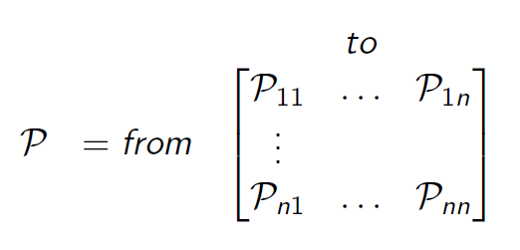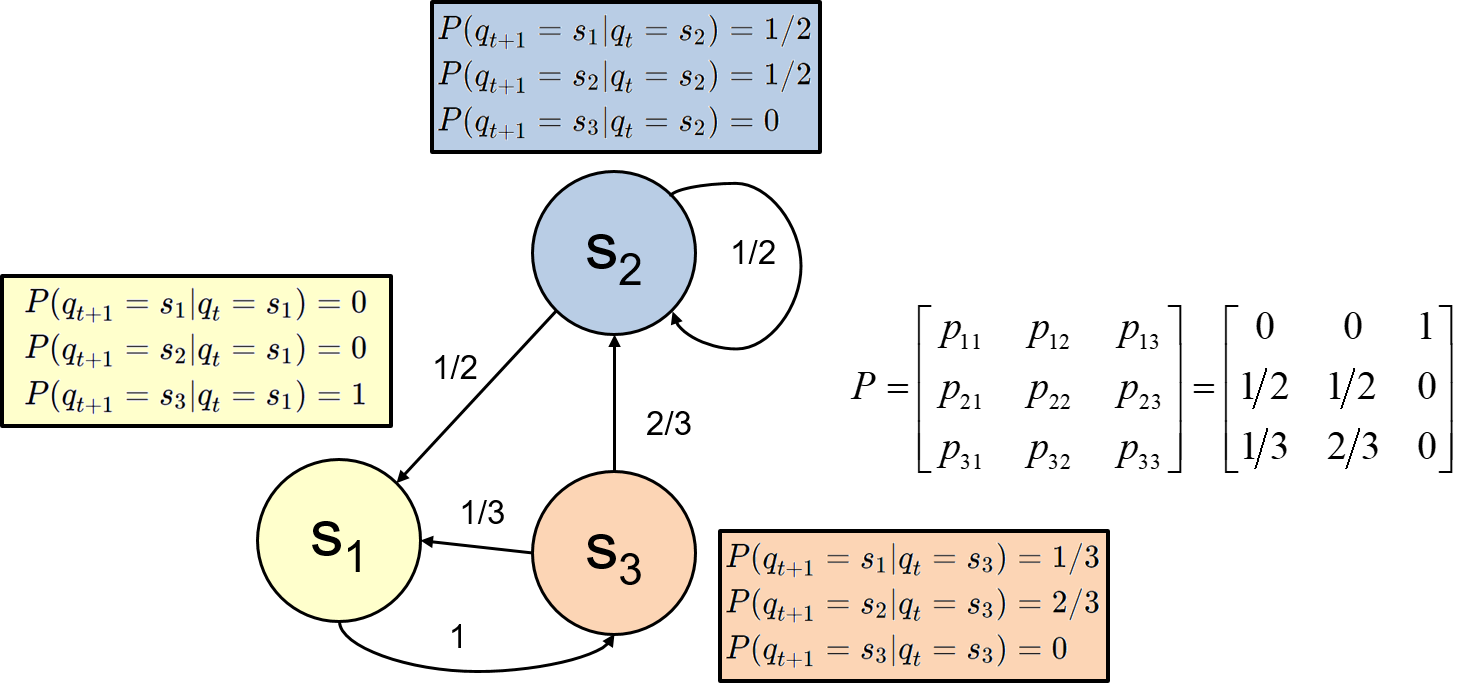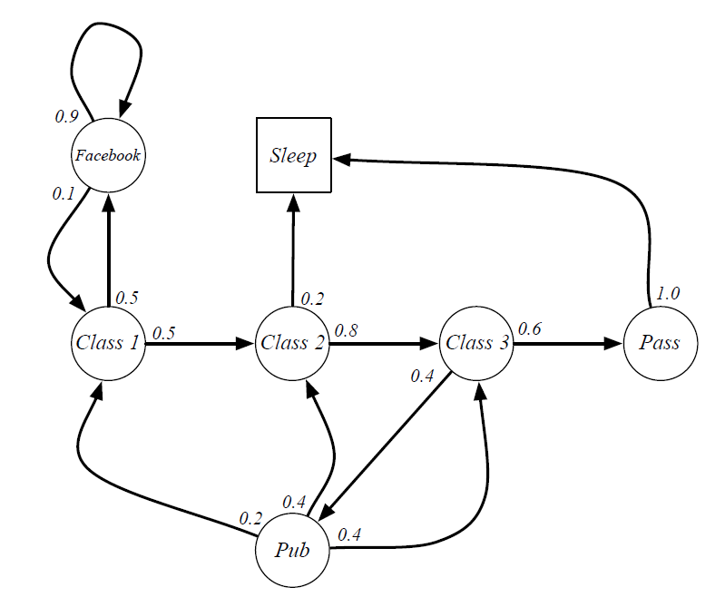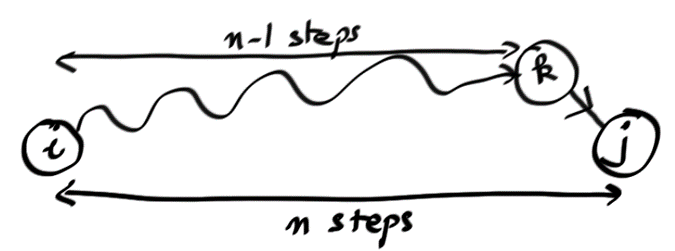Markov Chain

By Prof. Seungchul Lee
http://iai.postech.ac.kr/
Industrial AI Lab at POSTECH

# 1. Markov Process (or Chain)¶

## 1.1. Sequential Processes¶

• Most classifiers ignored the sequential aspects of data
• Consider a system which can occupy one of $N$ discrete states or categories $q_t \in \{S_1,S_2,\cdots,S_N\}$
• We are interested in stochastic systems, in which state evolution is random
• Any joint distribution can be factored into a series of conditional distributions

$$p(q_0,q_1,\cdots,q_T ) = p(q_0) \; p(q_1 \mid q_0) \; p( q_2 \mid q_1 q_0 ) \; p( q_3 \mid q_2 q_1 q_0 ) \cdots$$

Amost impossible to compute !!

$$p(q_0,q_1,\cdots,q_T ) = p(q_0) \; p(q_1 \mid q_0) \; p( q_2 \mid q_1 ) \; p( q_3 \mid q_2 ) \cdots$$

Possible and tractable !!

## 1.2. Markov Process¶

• (Assumption) for a Markov process, the next state depends only on the current state:

$$p(q_{t+1} \mid q_t,\cdots,q_0) = p(q_{t+1} \mid q_t)$$

• More clearly

$$p(q_{t+1} = s_j \mid q_t = s_i) = p(q_{t+1} = s_j \mid q_t = s_i,\; \text{any earlier history})$$

• Given current state, the past does not matter
• The state captures all relevant information from the history
• The state is a sufficient statistic of the future

## 1.3. State Transition Matrix¶

• For a Markov state 𝑠 and successor state 𝑠′, the state transition probability is defined by

$$P_{ss'} = P\left[S_{t+1} = s' \mid S_t = s \right]$$

• State transition matrix $P$ defines transition probabilities from all states $s$ to all successor states $s'$.Example: Markov chhain episodes

• sample episodes starting from $S_1$
In :
import numpy as np

P = [[0, 0, 1],
[1/2, 1/2, 0],
[1/3, 2/3, 0]]

In :
print(P[:])

[0.5, 0.5, 0]

In :
s = np.random.choice(3, 1, p = P[:])
print(s)

1

In :
# sequential processes
# sequence generated by Markov chain
# S1 = 0, S2 = 1, S3 = 2

# starting from 0
x = 0
S = []
S.append(x)

for i in range(20):
x = np.random.choice(3, 1, p = P[x][:])
S.append(x)

print(S)

[0, 2, 1, 1, 1, 1, 1, 0, 2, 0, 2, 1, 0, 2, 1, 0, 2, 1, 1, 1, 1]


## 1.4. Markov Chain Components¶

It represents passive stochastic behavior

$\;\;$ 1) a finite set of $N$ states, $S = \{ S_1, \cdots , S_N \}$

$\;\;$ 2) a state transition probability, $P = \{ p_{ij} \}_{M \times M},\quad 1 \leq i,j \leq M$

$\;\;$ 3) an initial state probability distribution, $\pi = \{ \pi_i\}$

Example of Markov Chain

• Starting from $S_1$ = Class 1In :
P = [[0, 0.5, 0, 0, 0, 0.5, 0],
[0, 0, 0.8, 0, 0, 0, 0.2],
[0, 0, 0, 0.6, 0.4, 0, 0],
[0, 0, 0, 0, 0, 0, 1],
[0.2, 0.4, 0.4, 0, 0, 0, 0],
[0.1, 0, 0, 0, 0, 0.9, 0],
[0, 0, 0, 0, 0, 0, 1]]

# sequential processes
# sequence generated by Markov chain
# [C1 C2 C3 Pass Pub FB Sleep] = [0 1 2 3 4 5 6]

name = ['C1','C2','C3','Pass','Pub','FB','Sleep']

# starting from 0
x = 0
S = []
S.append(x)

for i in range(5):
x = np.random.choice(7, 1, p = P[x][:])
S.append(x)

print(S)

[0, 5, 5, 5, 5, 5]

In :
episode = []

for i in S:
episode.append(name[i])

print(episode)

['C1', 'FB', 'FB', 'FB', 'FB', 'FB']


# 2. Chapman-Kolmogorov Equation¶

• (1-step transition probabilities) For a Markov chain on a finite state space, $S = \{ S_1, \cdots , S_N \}$, with transition probability matrix $P$ and initial distribution $\pi = \{ \pi_i^{0} \}$ (row vector) then the distribution of $X(1)$ is given by• (2-step transition probabilities) For a Markov chain on a finite state space, $S = \{ S_1, \cdots , S_N \}$, with transition probability matrix $P$ and initial distribution $\pi = \{ \pi_i^{0} \}$ (row vector) then the distribution of $X(2)$ is given by• (n-step transition probabilities) For a Markov chain on a finite state space, $S = \{ S_1, \cdots , S_N \}$, with transition probability matrix $P$ and initial distribution $\pi = \{ \pi_i^{0} \}$ (row vector) then the distribution of $X(n)$ is given by• $P^n$: n-step transition probabilities
• Key recursion
$$p_{ij}(n) = \sum_{k=1}^{N} p_{ik}(n-1) p_{kj}(1), \qquad i \rightarrow k \; \text{and}\; k \rightarrow j \;\text{imply}\; i \rightarrow j$$In :
# state probability distribution

P = [[0, 0, 1],
[1/2, 1/2, 0],
[1/3, 2/3, 0]]

u = [0, 1, 0]

P = np.asmatrix(P)
u = np.asmatrix(u)

for i in range(100):
u = u*P

print(u)

[[0.3 0.4 0.3]]

In :
u = [0, 1, 0]

u = u*P**10
print(u)

[[0.3000217  0.39989632 0.30008198]]


# 3. Stationary Distribution¶

• Does $p_{ij}(n)=P[X_n = j \mid X_0 = i]$ converge to some $\pi_j$ ?
• Take the limit as $n \rightarrow \infty$
$$p_{ij}(n) = \sum_{k=1}^{N} p_{ik}(n-1) p_{kj}$$$$\pi_j = \sum_{k=1}^{N} \pi_k p_{kj}$$
• Need also $\sum_j \pi_j = 1$
$$\pi = \pi P$$
In :
# eigenvalue = 1 and associated eigenvector

d, v = np.linalg.eig(P.T)

print(d)

[-0.25+0.32274861j -0.25-0.32274861j  1.  +0.j        ]

In :
v[:,2]/np.sum(v[:,2])

Out:
matrix([[0.3-0.j],
[0.4-0.j],
[0.3-0.j]])
In :
%%javascript
\$.getScript('https://kmahelona.github.io/ipython_notebook_goodies/ipython_notebook_toc.js')